# Welcome to Mental Math

Mental Math is a 501(c)3 nonprofit organization that focuses on the development of high quality math learning programs, worksheets and Practice work books. Our specialized materials cover all math concepts for children from pre-K through middle school.

Mental Math is an after-school supplementation program that aims to improve the mathematical skills and understanding of both struggling and talented students.

Our highly regarded team of math specialists have created and specially designed math worksheets and books, so students can start from their own level of learning ability. From here, they can gradually move to higher levels through a step-by-step hands-on learning process.

## Time Mental Math by Level

Starting from pre-k (or even before going to school) to level 10, these carefully crafted and timed mental math worksheets allow students to learn maths, starting at the appropriate difficulty for their competence. This provides each student and child the unique ability to find their exact starting point and then take the step-by-step process to gradually increase their skills and ability, and eventually master the mathematical concepts.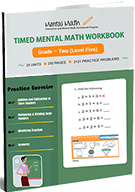### Mental Math Level Five

See all units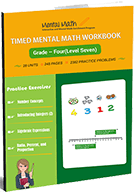### Mental Math Level Seven

Numeration Review, Multiplication, Division and Fraction

See all units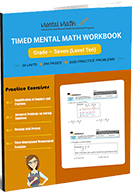### Mental Math Level Ten

Numeration Review, Multiplication, Division and Fraction

See all units

## Time Worksheets by Topics

Each specially designed timed worksheet for each topics (addition, subtraction, multiplication, division, fraction, decimal, integer, and equation), allows students to start problem solving at their own ability level. Students will gradually move step-by-step until they master every math concept.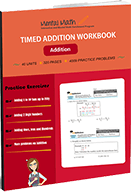Adding up to 5 digit Numbers and 3 Numbers

See all units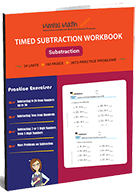### Timed Subtraction Worksheets

Subtracting 4 to 1-digit Numbers form 4 digit Numbers

See all units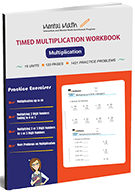### Timed Multiplication Worksheets

Multiplication Table of 2 through 10 and Multiplication by Thousands

See all units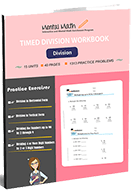### Timed Division Worksheets

Dividing up to 4 or more digit Numbers by 2 or 3-digit Numbers

See all units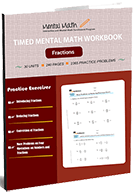### Timed Fractions Worksheets

Fraction Concepts and Four Operations on Fractions

See all units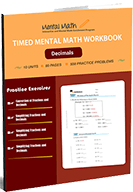### Timed Decimals Worksheets

Decimal Concept and Four Operations on Decimals

See all units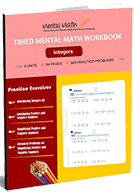### Timed Integers Worksheets

Four Operations on integers and their Simplification

See all units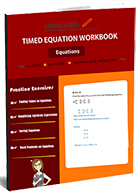### Timed Equation Worksheets

Evaluate Algebraic Expressions and Solve Equations

See all units